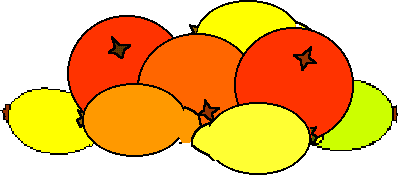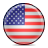#### You may also like### Exploring Wild & Wonderful Number Patterns

EWWNP means Exploring Wild and Wonderful Number Patterns Created by Yourself! Investigate what happens if we create number patterns using some simple rules.### Sending Cards

This challenge asks you to investigate the total number of cards that would be sent if four children send one to all three others. How many would be sent if there were five children? Six?### Dice and Spinner Numbers

If you had any number of ordinary dice, what are the possible ways of making their totals 6? What would the product of the dice be each time?

# Oranges and Lemons

##### Age 7 to 11Challenge Level

On the table there is a pile of oranges and lemons that weighs exactly one kilogram.The oranges all weigh $130$ grams. The lemons are also all the same weight, which is less than $\frac{2}{3}$ of the weight of an orange.

There are twice as many lemons as oranges in the pile.

How many lemons are there and how much does each one weigh?

(If you prefer to do this using pounds and ounces go here)